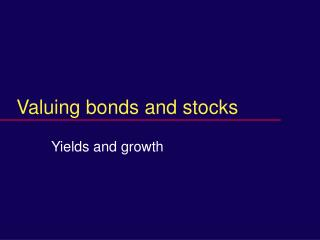Download PresentationValuing bonds and stocksValuing bonds and stocks - PowerPoint PPT Presentation

Download PresentationValuing bonds and stocks
An Image/Link below is provided (as is) to download presentation

Download Policy: Content on the Website is provided to you AS IS for your information and personal use and may not be sold / licensed / shared on other websites without getting consent from its author. While downloading, if for some reason you are not able to download a presentation, the publisher may have deleted the file from their server.

- - - - - - - - - - - - - - - - - - - - - - - - - - - E N D - - - - - - - - - - - - - - - - - - - - - - - - - - -
Presentation Transcript

1. Valuing bonds and stocks Yields and growth

2. Exam (sub) question • r = 6%, compounded monthly. • Save \$100 at the end of each month for 10 years. • Final value, in dollars of time 120?

3. Answer in two steps • Step 1. Find PDV of the annuity. • .005 per month • 120 months • PVAF = 90.073451 • PVAF*100 = 9007.3451 • Step 2. Translate to money of time 120. • [(1.005)^120]*9007.3451 = 16387.934

4. Present value of annuity factor

5. Example: Cost of College • Annual cost = 25000 • Paid when? • Make a table of cash flows

6. Timing • Obviously simplified

7. Present value at time zero • 25+25*PVAF(.06,3) • =91.825298

9. Saving for college • Start saving 16 years before matriculation. • How much each year? • Make a table.

10. The college savings problem

11. Solution outlined • Target = 91.825 dollars of time 16. • Discount to dollars of time 0. • Divide by (1.06)16 • Result 36.146687… , the new target • PV of savings =C+C*PVAF(.06,16) • Equate and solve for C.

12. Numerical Solution • PV of target sum = 36.146687 • PV of savings = C+C*10.105895 • Solve C*11.105695 = 36.14667 • C = 3.2547298

13. Confirmation in an excel spread sheet.

14. Finish here 1/17/06

15. Apply the formula to a Bond This is a bond maturing T full years from now with coupon rate 2C/1000. C is the coupon payment.

16. Yield • Yield is a market rate now. • Coupon rate is written into the bond. • It is near the market rate when issued. • Yield and coupon rate are different.

17. Given the yield, r • Yield r for a bond with semi-annual coupons means r/2 each 6 months. • Value of the bond that matures in T years is • P = C*PVAF(r/2,2T) + 1000/(1+r/2)2T

18. Given the price of the bond, P • Yield is the r that satisfies the valuation equation • P=C*PVAF(r/2,2T) + 1000/(1+r/2)2T

19. A typical bond

20. Value at yield of 5% • Pure discount bond (the 1000): Value =1000/(1.025)3=928.599… • Strip: ( the coupon payments)60*(1/.025)(1-1/(1.025)3) • =171.3614… • Total market value of bond =1099.96

21. Facts of bonds • They are called, • at the option of the issuer when interest rates fall. • or retired in a sinking fund, • as required to assure ultimate repayment.

22. More Facts • Yield > coupon rate, bond sells at a discount (P<1000) • Yield < coupon rate, it sells at a premium(P>1000)

23. Growing perpetuities • Thought to be relevant for valuing stocks • Present value of growing perpetuity factor PVGPF • g = growth rate (decimal) • r = interest rate (decimal) • PVGPF(r,g) = 1/(r-g)

24. Growing perpetuity

25. Riddle • What if the growth rate is above the discount rate? • Formula gives a negative value. • Correct interpretation is infinity.

26. More riddle: market response • An investment with growth rate above the interest rate. • Others copy the investment until competition drives the growth rate down • or until … • the opportunity drives the interest rate up.

27. Review question • A bond has a coupon rate of 8%. • The maturity is 10 years from now. • It sells today at par, that is, for \$1000. • What is the yield? • Prove it.

28. Answer one • yield = coupon rate. • You must know that.

29. Answer two: proof • 1000/(1.04)20+ 40*(1/.04)[1-1/(1.04)20] = 456.3869462+543.6130537 = 1000

30. Answer two: deeper proof • 1000/(1.04)20+ 40*(1/.04)[1-1/(1.04)20] • 1000/(1.04)20 + 1000-1000/(1.04)20 • End terms cancel. Answer = 1000.

31. Growing perpetuity

32. Example: share of stock • The market expects a dividend of \$4 in one year. • It expects the dividend to grow by 5% per year • The discount rate for such firms is 16%. • What is the price of a share?

33. Solution • P=4*(1/(.16-.05)) • =36.3636...

34. Decomposition of value • Absent growth, as a cash cow,value = 4*(1/.16) • = 25. • Remaining value of 36.3636… - 25 is net present value of growth opportunities (NPVGO). • =11.3636...

35. Example: whole firm • The market expects \$30M in one year • and growth of 2% thereafter. • Discount rate = 17%. • Value of the firm is \$200M. • That is 30M*(1/(.17-.02))

36. continued • A new line of business for the firm is discovered. • The market expects \$20M in a year, • with growth at 7% thereafter. • Value of the new growth opportunity is \$200M (at r = 17%).

37. Whole value:400M = 200M + 200M • Note that the value is gross, not net. • Share price? • Divide by the number of shares.

38. Why should we be skepticalabout the PV growing perpetuity • The value is coming from far distant years.